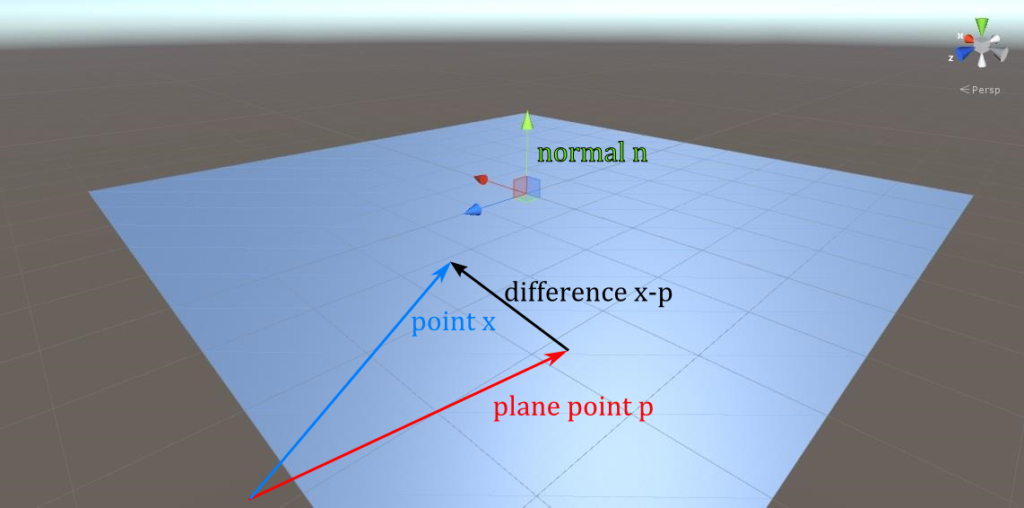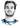# Howto Raytracer: Ray / Plane Intersection Theory

Categories:

In this tutorial I will derive how to calculate the intersection of a ray and a plane.

As already stated in my ray / sphere intersection howto, a ray $r(t)$ can be represented by a point on the ray $e$ and the ray’s direction $d$: $r(t)=e + t d$. The set $R$ of all points on the ray is then given by: $R = \{r(t) \mid t \in \mathbb{R}\}$

Similarly, a plane $P$ can be represented by a point on the plane $p$ and by its normal $n$. So how do we characterize the points on a plane? The main insight is that given any two points $a,b$ on the plane, the vector from $a$ to $b$, i.e. $b-a$, lies itself inside the plane and is thus by definition of the plane’s normal $n$ perpendicular to it. To check for perpendicularity, we check if the dot product $(b-a)\cdot n$ is $0$. The set of the plane’s points $x$ is then given by $P = \{(x-p) \cdot n = 0 \mid x \in \mathbb{R}^3\}$To find the intersection of the ray and the plane now, we have to find the points that are in both sets. So we check if a point $r(t)$ on the ray also fullfills the plane equation:

\begin{aligned} (r(t) - p) \cdot n = 0 \\\\ (e + t d - p) \cdot n = 0 \\\\ e \cdot n + t d \cdot n - p \cdot n = 0 \\\\ t = \frac{p \cdot n - e \cdot n }{d \cdot n} \\\\ t = \frac{(p - e) \cdot n }{d \cdot n} \end{aligned}

So we just calculate $t$, plug it back in the ray’s equation $r(t)$ and end up with the hit point, unless the denominator $d \cdot n$ is $0$ in which case there is no intersection. Geometrically this corresponds to the ray and the plane being parallel.

Here’s some sample code that implements this collision test:

    public override RayTracer.HitInfo Intersect(Ray ray)
{
RayTracer.HitInfo info = new RayTracer.HitInfo();

Vector3 d = ray.direction;
float denominator = Vector3.Dot(d, normal);

if (Mathf.Abs(denominator) < Mathf.Epsilon) return info;      // direction and plane parallel, no intersection

float t = Vector3.Dot(center - ray.origin, normal) / denominator;
if (t < 0) return info;    // plane behind ray's origin

info.time = t;
info.hitPoint = ray.GetPoint(t);
info.normal = normal;
return info;
}

All in all, ray / plane intersection is rather easy if you ‘re working with inifinitely long planes. If your planes have a width and height, it gets drastically more complicated using just a normal to represent a plane, because this representation is ambiguous to rotations around the normal. However for planes with finite width/height this orientation is important and you would be better suited representing the plane by the (orthogonal) vectors that span it.

# Hi, I'm Christoph Michel 👋I'm a , , and .

I mostly do and help small to mid-sized teams from all over the world 🌎 on both short and long-term projects on an independent contractor basis.

I strive for efficiency ⏱️ and therefore track many aspects of my life.